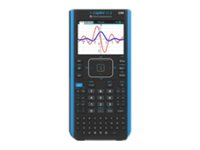BrowseBrowse by Category
Browse by Category
Help
•# Texas Instruments - Texas Instruments TI-Nspire CX II CAS Teacher Pack

Graphing calculator - USB - battery (pack of 10)

Texas Instruments
NSCXCAS2/TPK/2L1
0033317208432
N/A
New

## Price: \$2,123.86

Total Available: 97

## Specifications

Main Specifications
Product Description Texas Instruments TI-Nspire CX II CAS Teacher Pack - graphing calculator
Product Type Graphing calculator - LCD
Packaged Quantity 10 (specifications are for single item)
Interface USB
Basic Functions Addition, subtraction, multiplication, division
Scientific Functions Binary, complex numbers, advanced probability distribution, statistical analysis, linear regression, hypothesis testing, confidence intervals, matrix, logarithmic regression, exponential regression, power regression, differential, derivatives, inverse matrix, quadratic polynomial regression, cubic polynomial regression, quartic polynomial regression, median-median regression, logistic regression, sinusoidal regression, analysis of variance, determinant, transpose, chemical notation, symbolic units, binomial distribution, poisson distribution
Financial Functions Amortization, cash flow, Time Value of Money (TVM), Net Future Value (NFV), Modified Internal Rate of Return (MIRR)
Graphing Functions 3D graphing, polar, sequence, zoom, parametric plot, histogram, scatter plot, plot statistics, box and whisker plot, geometric figure graphing
Diagonal Size 3.5"
Color Support Color
Power Source Battery
General
Packaged Quantity 10 (specifications are for single item)
Product Type Graphing calculator
Interface USB
Power Source Battery
RAM 64 MB
Miscellaneous
Included Accessories 10 x batteries, 5 x unit-to-unit USB cables, 10 x USB cables, docking station
Warranty 1 year
Software
Software Included Computer Algebra System (CAS)
Display
Type LCD
Diagonal Size 3.5"
Color Support Color
Display Resolution 320 x 240 pixels
Features Backlighting
Functions